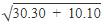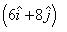Courses

# Test: Relative Motion

## 10 Questions MCQ Test Physics Class 11 | Test: Relative Motion

Description
This mock test of Test: Relative Motion for Class 11 helps you for every Class 11 entrance exam. This contains 10 Multiple Choice Questions for Class 11 Test: Relative Motion (mcq) to study with solutions a complete question bank. The solved questions answers in this Test: Relative Motion quiz give you a good mix of easy questions and tough questions. Class 11 students definitely take this Test: Relative Motion exercise for a better result in the exam. You can find other Test: Relative Motion extra questions, long questions & short questions for Class 11 on EduRev as well by searching above.
QUESTION: 1

Solution:
QUESTION: 2

Solution:
QUESTION: 3

### Rain is falling vertically with the speed of 30 m/s. A man rides a bicycle with the speed of 10 m/s in east to west direction. What is the direction in which he should hold the umbrella?

Solution:

When the man rides, he should put an umbrella in the opposite direction to which the drops are falling with respect to him.
Hence wrt man, the speed of rain drop isat an angle of tan-1
Hence he would put umbrella to the direction of tan-1

QUESTION: 4

The angular speed of a wheel of radius 0.50 m moving with the speed of 20 m/s is:

Solution:

Speed, v = 20 m/s
We know,
v = r ω
where,
ω = angular velocity
20 = 0.5 x ω
ω = 20/0.5 = 40 rad/sec

QUESTION: 5

In 5 seconds a body covers a distance of 50 m and in 10 seconds it covers 80 m with uniform acceleration. What is the distance traveled in 15 seconds?

Solution:

Earth rotates by the angle of 2 that is a complete rotation in 24hrs
Thus its angular speed, w = 2 / 24 x 60 x 60 rad/sec

QUESTION: 6

The angular speed of a flywheel making 210 revolutions per minute is:

Solution:

As the flywheel makes 210 revolution in a minute, and during each revolution the angular displacement of the flywheel  is 2π thus total angular distance covered is
210 × 22/7 × 2 = 1320 rad

QUESTION: 7

A boat is moving with a velocitywith respect to the ground. The water in the river is moving with a velocitywith respect to the ground. What is the relative velocity of boat with respect to river?

Solution:

Velocity of boat wrt river = velocity of boat wrt ground - velocity of river wrt ground
I.e. v = (6i + 8j) - (-6i - 4j)
= 12i + 12j

QUESTION: 8

The angular speed of the second hand of a watch is :

Solution:

QUESTION: 9

A monkey is running on the ground, next to a train, in the direction of the train. The speed of the monkey is 5km/h and the speed of the train is 100km/h. What is the velocity of the monkey with respect to the train?

Solution:

Assuming the direction of velocity of train to be positive, wrt train the speed of monkey will be
-100 + 5 = -95 kmph
I.. 95 kmph in direction opposite to the train.

QUESTION: 10

The angular speed of a wheel of radius 0.50 m moving with the speed of 20 m/s is:

Solution:

Speed, v = 20 m/s

We know,

v = r ω

where,

ω = angular velocity

20 = 0.5 x ω

ω = 20/0.5 = 40 rad/sec